Next: The paradox of antipodes Up: Relativistic time Previous: The modified twins paradox   Contents

Now we shall pass to the time paradox for moving systems. For "resolving" it, the Lorentz transformations are often used: they allow one to put in correspondence to one time instant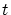the whole continuum of times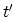. Note, that if we compare the time intervals, then the procedure of synchronizing the time reference point is unimportant. Let us have four clocks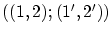, spaced similarly in pairs and synchronized in their own systems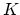and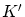(Fig. 1.3). The synchronization can, for example, be performed by an infinitely remote source located on the axis perpendicular to the plane of all four clocks (it will be further outlined in the subsection on "establishing the universal absolute time").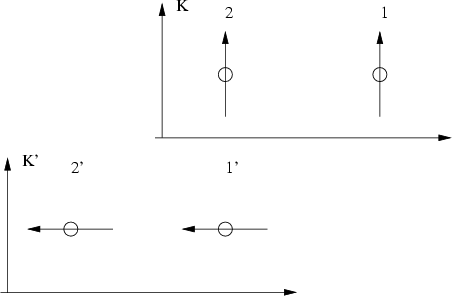Then for any intervals we have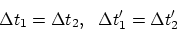(1.1)

However, according to the Lorentz transformations formulas, from the point of view of observers in system(near the clocks), at the time of coincidence of clocks we have (Fig. 1.4):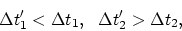(1.2)

i. e. inequality (1.2) contradicts equality (1.1). A similar contradiction with (1.1) occurs if the inequalities are written from the point of view of observers in system(near the clocks). Even the values of differences of time intervals will be different.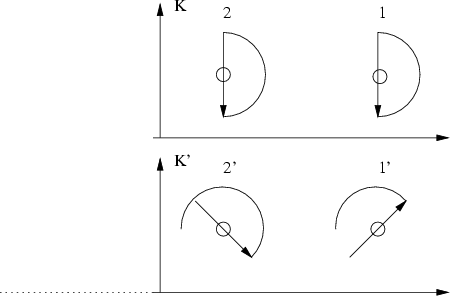Thus, these four observers will not be able to agree among themselves, when they meet at one point and discuss the results. Where then is the objectiveness of science?Next: The paradox of antipodes Up: Relativistic time Previous: The modified twins paradox   Contents
Sergey N. Arteha